Select Page

# Integrals Maths 12 Science CBSE Solutions for MCQ in English

Integrals Maths 12 Science CBSE Solutions for MCQ in English to enable students to get Solutions in a narrative video format for the specific question.

Expert Teacher provides Integrals Maths 12 Science CBSE Solutions for MCQ through Video Solutions in English language. This video solution will be useful for students to understand how to write an answer in exam in order to score more marks. This teacher uses a narrative style for a question from Integrals not only to explain the proper method of answering question, but deriving right answer too.

Please find the question below and view the Solution in a narrative video format.

Question:

Solution Video in English:

## Similar Questions from CBSE, 12th Science, Maths, Integrals

Question 1 : Evaluate :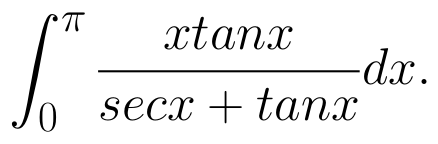(View Answer Video)

Question 2 : Find the integral of the function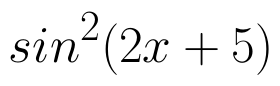. (View Answer Video)

Question 3 : Find :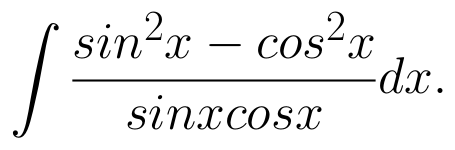(View Answer Video)

Question 4 :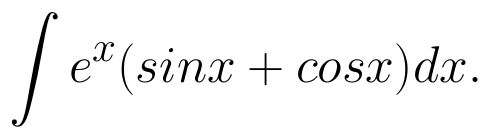(View Answer Video)

Question 5 : Find :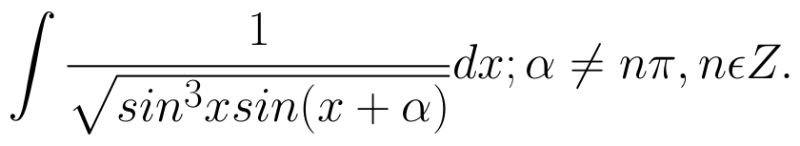(View Answer Video)

### Determinant

Question 1 : If area of a triangle is 35 sq unit with vertices (2, -6), (5, 4) and (k, 4), then k is, (View Answer Video)

Question 2 : If A =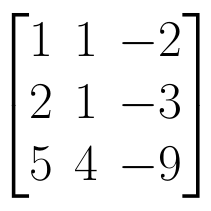, find | A |. (View Answer Video)

Question 4 : If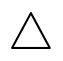=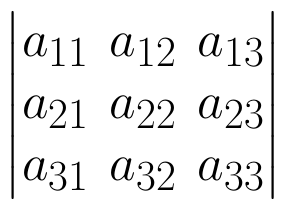and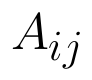is Cofactor of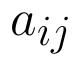, then the value ofis given by (View Answer Video)

Question 5 : If A is a symmetric matrix, then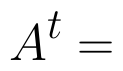? (View Answer Video)

### Three Dimensional Geometry

Question 1 : Find the distance of the point (2, 12, 5) from the point of intersection of the lines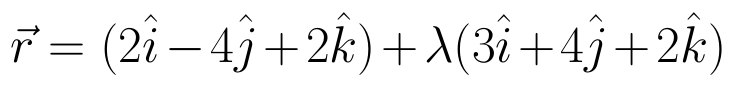and the plane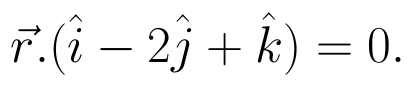(View Answer Video)

Question 2 : Find the distance of the point (1, -2, 3) from the plane x-y+z=5 measured parallel to the line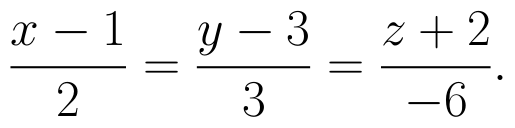(View Answer Video)

Question 3 : Find the distance of the plane : 3x - 4y + 12z = 3 from the origin. (View Answer Video)

Question 4 : What is the distance of the point (p, q, r) from the x-axis ? (View Answer Video)

Question 5 : If a line has direction ratios 2, -1, -2, then what are its direction cosines? (View Answer Video)

### Application of Integrals

Question 1 : Find the area of the region in the first quadrant enclosed by x-axis, line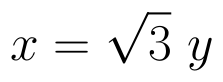and the circle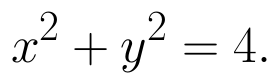(View Answer Video)

Question 2 : Using the method of integration, find the area of the region bounded by the lines 3x - 2y + 1 = 0, 2x + 3y -21 = 0 and x - 5y + 9 = 0. (View Answer Video)

Question 3 : Area lying in the first quadrant and bounded by the circle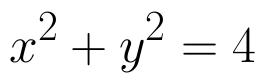and the lines x =0 and x = 2 is (View Answer Video)

Question 4 : Find the area of the circle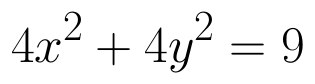which is interior to the parabola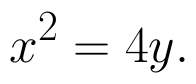(View Answer Video)

Question 5 : Using integration, find the area of the region bounded by the triangle whose vertices are (-1, 2), (1, 5) and (3, 4). (View Answer Video)